Next: Finding Good Matchings Up: Generating the Edit Previous: Aligning Children

## The Complete Algorithm

We now present the complete algorithm to compute a minimum cost edit script E conforming to a given matching M between trees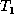and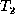. In the algorithm, we combine the first four phases of Section 4.1 (the update, insert, align, and move phases) into one breadth-first scan on. The delete phase requires a post-order traversal of(which visits each node after visiting all its children). The order in which the nodes are visited and the edit operations are generated is crucial to the correctness of the algorithm. (For example, an insert may need to precede a move, if the moved node becomes the child of the inserted node.) The algorithm applies the edit operations toas they are appended to the edit script E. When the algorithm terminates,is isomorphic to. The algorithm also uses a matching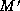that is initially M, and adds matches to it so thatis a total matching when the algorithm terminates. As mentioned earlier, we assume without loss of generality that the roots ofandare matched in M.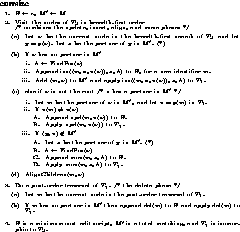Figure 8: Algorithm EditScript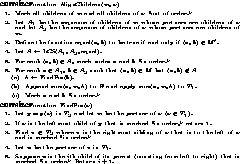Figure 9: Functions AlignChildren and FindPos

The algorithm is shown in Figure 8. It uses two procedures, AlignChildren and FindPos, shown in Figure 9. The claims made by the two statements in Algorithm EditScript that are marked with (*) are substantiated in [CRGMW95], where it is also proved that Algorithm EditScript generates a minimum cost edit script conforming to the given matching M.

Let us now consider the running time of this algorithm. We first define the notion of misaligned nodes. Suppose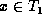and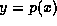. A move of the form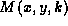for some k is called an intra-parent move of node x; such moves are generated in the align phase of the algorithm. The number of misaligned nodes ofwith respect tois the minimum number of intra-parent moves among all minimum cost edit scripts. We can show [CRGMW95] that the running time of Algorithm EditScript is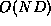, where N is the total number of nodes inandand D is the total number of misaligned nodes. (Note that D is typically much smaller than N.)Next: Finding Good Matchings Up: Generating the Edit Previous: Aligning Children

Sudarshan S. Chawathe
Wed Jun 19 08:22:34 PDT 1996